# Internal Division

Go back to  'Similar-Triangles'

You are given a line segment AB: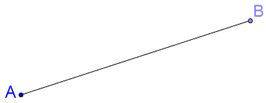How will you divide it in a given (rational) ratio? Suppose that you are asked to internally divide AB in the ratio 3:4. This means that you have to find a point C on AB such that AC:CB = 3:4, as shown below: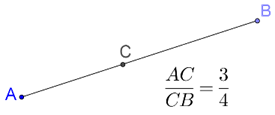How will you geometrically locate point C? This is the problem of interval division of a given line segment in a given ratio. We are going to use the BPT to guide our construction.

Step 1: Draw any ray AX inclined at an arbitrary angle to AB, and mark 3 + 4 = 7 equal intervals on AX, as shown below: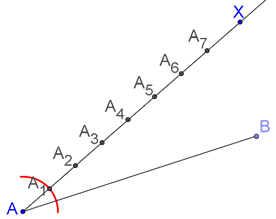This can be done easily using a compass. In the figure, we have shown one arc (to construct the first interval)

Step 2: Join the last point (in this case, A7) to B: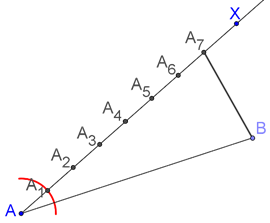Step 3: Through A3, draw a parallel to A7B. The point of intersection of this parallel with AB is the required point C: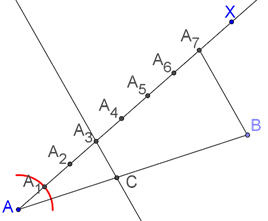The proof is straightforward. We have A3C || A7B. Using the BPT, we have:

$\frac{{A{A_3}}}{{{A_3}{A_7}}} = \frac{3}{4} = \frac{{AC}}{{CB}}$

Using this approach, we can divide any given segment in an arbitrary rational ratio. As an exercise, construct some line segments and divide them in the following ratios: 3:5, 2:3, 1:7, 6:7, 2:11.

Learn math from the experts and clarify doubts instantly

• Instant doubt clearing (live one on one)
• Learn from India’s best math teachers
• Completely personalized curriculum